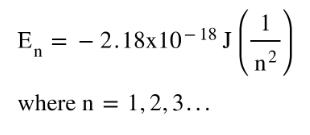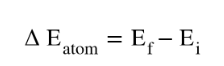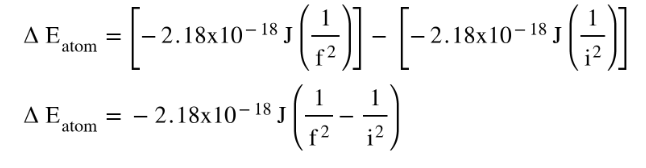# Problem: Before quantum mechanics was developed, Johannes Rydberg developed an equation that predicted the wavelengths (λlambda) in the atomic spectrum of hydrogen: 1/λ = R(1/m2 - 1/n2). In this equation R is a constant and m and n are integers. Use the quantum-mechanical model for the hydrogen atom to derive the Rydberg equation.

###### FREE Expert Solution
• For an H atom, the energy of an electron in level n is represented as:• An electron jumping from higher energy level f to lower energy level i, the energy released by atom will appear as:• Use the equation of En to find the ΔE.83% (62 ratings)###### Problem Details

Before quantum mechanics was developed, Johannes Rydberg developed an equation that predicted the wavelengths (λ) in the atomic spectrum of hydrogen: 1/λ = R(1/m2 - 1/n2). In this equation R is a constant and m and n are integers. Use the quantum-mechanical model for the hydrogen atom to derive the Rydberg equation.